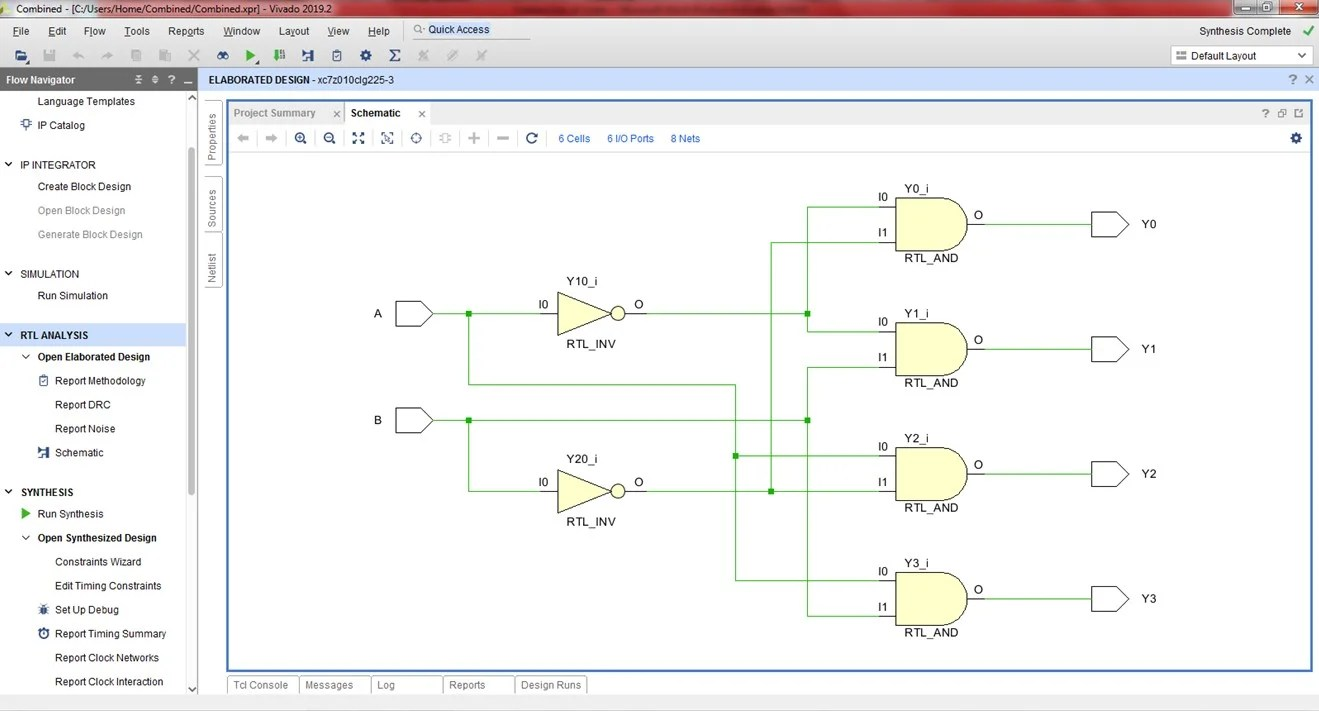View Course Path

# VHDL code for decoder using dataflow method – full code and explanation

Now that we have written the VHDL code for an encoder, we will take up the task of writing the VHDL code for a decoder using the dataflow architecture. As customary in our VHDL course, first, we will take a look at the logic circuit of the decoder. Then we will take a look at its logic equation. And then, we will understand the syntax. Once we have written the code to generate the netlist (RTL synthesis), we will write the testbench to verify our design, generate an RTL schematic and the simulation waveforms.

Contents

## Explanation of the VHDL code for decoder using dataflow method. How does the code work?

A decoder is a combinational logic circuit that does the opposite job of an encoder. Essentially, it takes in a coded binary input and decodes it to give a higher number of outputs. Check out the working of a decoder in-depth over here.

In this post, we are writing the VHDL code for a 2:4 decoder using the dataflow modeling architecture. This means that we need its logic equations.

If you are familiar with digital electronics, we usually get our logic equations from the truth table of the said circuit. But first, since we have already designed this circuit in our digital electronics course, we will take a look at the circuit of the 2:4 decoder.Honestly, we don’t care about the internal gates right now. The purpose right now is to find the number and type of ports. Because we will need that data to write the entity-architecture pair for the VHDL code. As we can see, the 2:4 decoder has two input ports and four output ports.

Cool.

Let’s move on to finding its logic equation now. We are going to need the truth table for that.

### Truth table for a 2:4 decoder

 A B Y3 Y2 Y1 Y0 0 0 0 0 0 1 0 1 0 0 1 0 1 0 0 1 0 0 1 1 1 0 0 0

From this truth table, we get the following logic equations for the outputs.

Y0 = A’B’

Y1 = A’B

Y2 = AB’

Y3 = AB

Now let’s start writing the code.

Include the IEEE libraries (IEEE, 1164, arithmetic, and unsigned).

Next up is the entity-architecture declaration.

Within the `entity`, we will declare the ports. Architecture is one of the three VHDL modeling styles that we will be using to write the code. Here we are using the dataflow architecture.

Let’s call this entity as `DECODER_SOURCE`. We have two input ports and four output ports. We will define them as standard logic and not vectors. Why? Because we are using an equation for each one of them, and they need to be able to be addressed individually.

Here’s what our entity-architecture pair will look like:

```entity DECODER_SOURCE is
Port ( A,B : in  STD_LOGIC;
Y3,Y2,Y1,Y0 : out  STD_LOGIC);
end DECODER_SOURCE;

architecture dataflow of DECODER_SOURCE is

begin```

And now the actual program. All we have to do is define and assign the relationship between our ports using the assignment operator. Don’t forget to end the architecture with the end command.

```Y0 <= ((not A)and(not B));
Y1 <= ((not A) and B);
Y2 <= (A and (not B));
Y3 <= (A and B);```

## VHDL code for decoder using dataflow method

2:4 DECODER DATAFLOW

```library IEEE;
use IEEE.STD_LOGIC_1164.ALL;
use IEEE.STD_LOGIC_ARITH.ALL;
use IEEE.STD_LOGIC_UNSIGNED.ALL;

entity DECODER_SOURCE is
Port ( A,B : in  STD_LOGIC;
Y3,Y2,Y1,Y0 : out  STD_LOGIC);
end DECODER_SOURCE;

architecture dataflow of DECODER_SOURCE is

begin

Y0 <= ((not A)and(not B));
Y1 <= ((not A) and B);
Y2 <= (A and (not B));
Y3 <= (A and B);

end dataflow;

```

## Testbench

Writing a VHDL testbench is an essential skill as we have learnt in this VHDL course. Let’s use a testbench with a process statement for this decoder.

```library IEEE;
use IEEE.STD_LOGIC_1164.ALL;
use IEEE.STD_LOGIC_ARITH.ALL;
use IEEE.STD_LOGIC_UNSIGNED.ALL;

entity decoder_tb is
end entity;

architecture tb of decoder_tb is
component DECODER_SOURCE is
Port ( A,B : in STD_LOGIC;
Y3,Y2,Y1,Y0 : out STD_LOGIC);
end component;

signal A, B, Y3, Y2, Y1, Y0 : STD_LOGIC;

begin

uut: DECODER_SOURCE port map(
A => A, B => B,
Y0 => Y0, Y1 => Y1,
Y2 => Y2, Y3 => Y3);

stim: process
begin

A <= '0';
B <= '0';
wait for 20 ns;

A <= '0';
B <= '1';
wait for 20 ns;

A <= '1';
B <= '0';
wait for 20 ns;

A <= '1';
B <= '1';
wait for 20 ns;

wait;

end process;
end tb;```

## RTL Schematic## Simulation WaveformEdit: Post updated with the testbench, RTL Schematic, and Simulation Waveform by Deepak Joshi.

This site uses Akismet to reduce spam. Learn how your comment data is processed.

Top